# Different types of Attention in Neural Networks

Reading through various attention related papers gives an interesting perspective on how researchers have used attention mechanisms for various tasks and how the thinking has evolved. This is a quick overview of such a study that will give a sense of how we could tweak and use attention based architectures for our own tasks.

In an earlier post on “Introduction to Attention” we saw some of the key challenges that were addressed by the attention architecture introduced there (and referred in Fig 1 below). While in the same spirit, there are other variants that you might come across as well. Among other aspects, these variants differ on are “where” attention is used ( standalone, in RNN, in CNN etc) and “how” attention is derived (global vs local, soft vs hard etc).This post is a brief listing of some of the variants.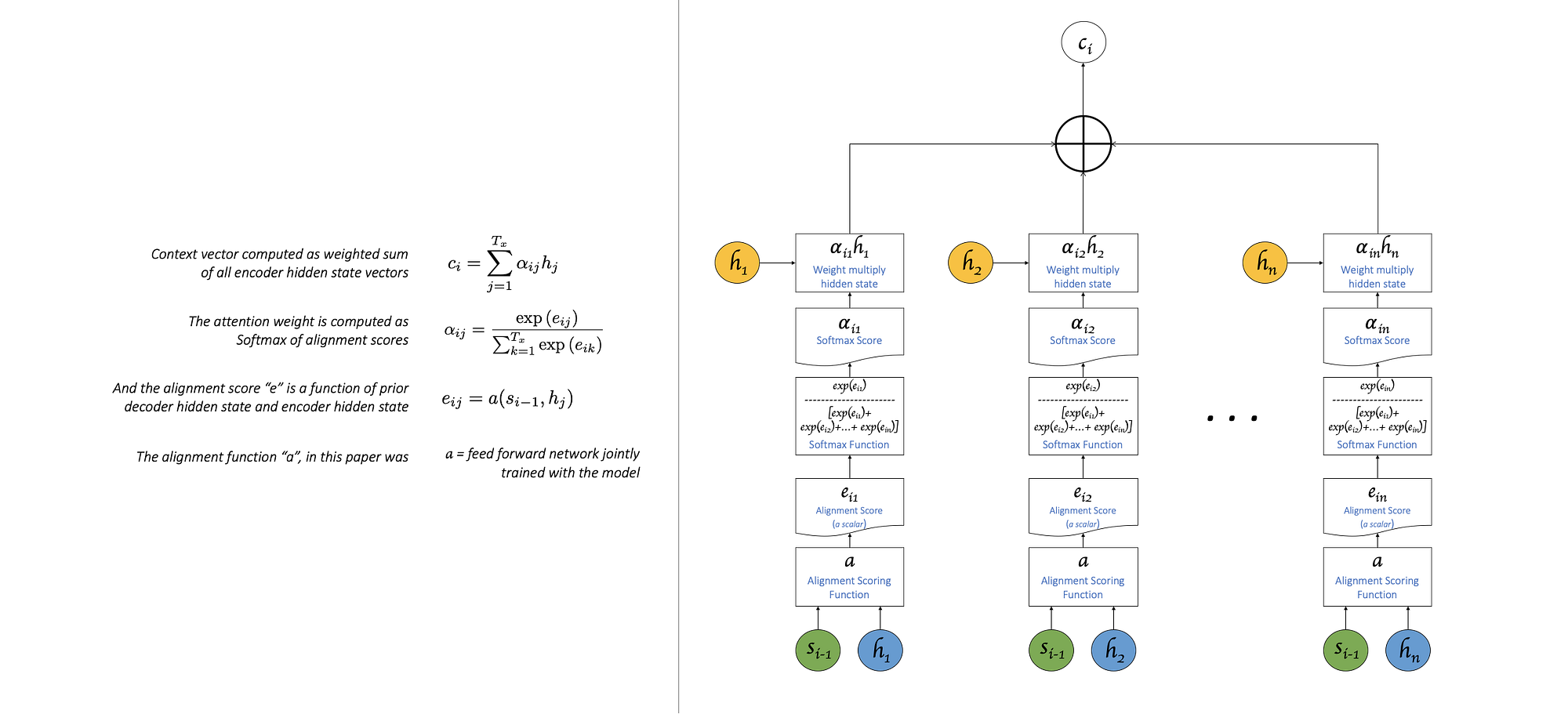Fig 1: From “Introduction to Attention”, based on paper by Bahdanau et al.

Disclaimer 1: The idea here is just to get a sense of how attention mechanisms are leveraged in solutions proposed in different papers. So the focus will be less on the type of task the paper was trying to solve and more on the usage of attention mechanisms in the solution.

Disclaimer 2: There is no rigor or reason behind why these papers/variants were chosen. This list is just a product of random scouting and top search hits.

Disclaimer 3: Self attention and Transformers deserve a separate post (truly, I lost steam for the day) and are not touched upon here.

Global Attention vs Local attention

Global attention is the same as what was explored in the “Introduction to Attention” post. It is when we use ALL encoder hidden states to define the attention based context vector for each decoder step. But as you might have guessed, this could become expensive.

Local attention on the other hand attends to only a few hidden states that fall within a smaller window. This window is centered around the “p”th encoder hidden state and includes “D” hidden states that appear on either side of “p”. So that makes the length of this window, i.e. the number of hidden states considered, 2D+1. Monotonic alignment is when p is simply set to be the same as the decoder position (5th output will have p = 5, and if D = 2, the attention will be only on 3,4,5,6,7 hidden states). Predictive alignment is when “p” is defined as a function of the decoder hidden state ht (paper uses S · sigmoid(vp⊤ tanh(Wpht))) and the parameters of this function are jointly learnt by the model.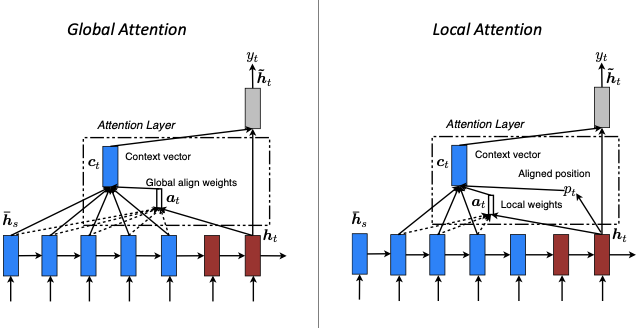Fig 2: Global vs Local Attention as defined by Luong et al. here

Hard vs Soft attention

Referred by Luong et al. in their paper and described by Xu et al. in their paper, soft attention is when we calculate the context vector as a weighted sum of the encoder hidden states as we had seen in the figures above. Hard attention is when, instead of weighted average of all hidden states, we use attention scores to select a single hidden state. The selection is an issue, because we could use a function like argmax to make the selection, but it is not differentiable (we are selecting an index corresponding to max score when we use argmax and nudging the weights to move the scores a little as part of backprop will not change this index selection) and therefore more complex techniques are employed. Note, the paper uses hard attention in an image captioning context, so “the encoder hidden states” are really the “feature vectors” generated by a CNN.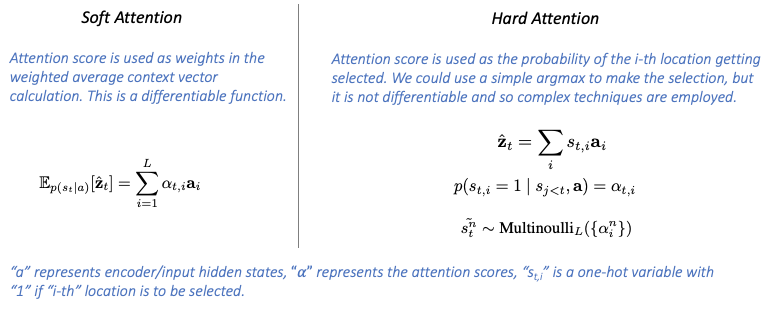Fig 3: Soft vs Hard Attention as defined by Xu et al.

Latent attention

I stumbled upon this paper presented by Chen et al. which deals with translating natural language sentences in to “If-Then” programs. i.e., given a statement like “Post your Instagram photos to Tumblr”, the network should predict the most relevant words describing the trigger (“Instagram photos”) and action (Tumblr) which would then help arrive at the corresponding labels (trigger=Instagram.Any_new_photo_by_you, action=Tumblr.Create_a_photo_post).

How could we apply attention to arrive at this? Let’s look at another example, “Post photos in your Dropbox folder to Instagram”. Compared to the previous one, here “Instagram” is the most relevant for action and “Dropbox” is the trigger. The same word can be either the trigger or the action. So determining what role the word plays require us to investigate how the prepositions like “to” are used in such sentences. The paper introduces a “Latent Attention” model to do this.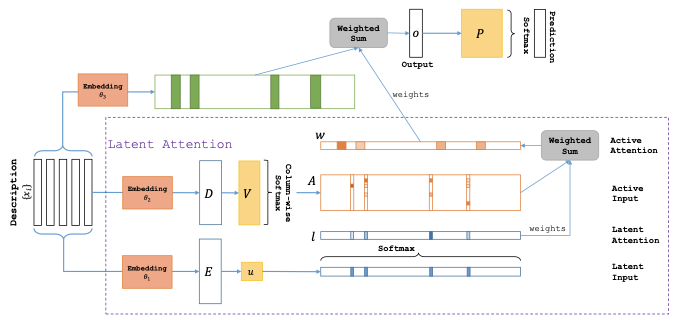Fig 4: “Latent Attention” presented by Chen et al. in this paper

A “J” dimensional “Latent attention” vector is prepared — each dimension here represents a word, and the softmax gives a sense of relative importance across the words in the vector.

1. Input sequence is of length “J” (i.e “J” words). Each word represented by a “d” dimensional embedding vector. The entire sequence is therefore a d x J matrix.
2. The product of this matrix with a trainable vector “u” of length “d” is computed, with a softmax over it. This Gives the “Latent attention” vector of length “J”

Next, “Active Attention” is prepared similar to above, but instead of using a “d” dimensional vector like “u”, a “d x J” dimensional trainable matrix V is used, resulting in a “J x J” Active attention matrix. Column-wise softmax is done between the dimensions of each word.

The “Active Weights” are then computed as the product of these two. Another set of word embeddings are then weighted by these “Active Weights” to derive the output which is the softmaxed to arrive at the predictions.

To me, the derivation of the active weights as a product of vectors representing each word in the input and the latent attention vector that represents the importance across the words is a form of “self attention”, but more on self attention later.

Attention Based Convolutional Neural Network

In this paper Yin et al presented ABCNN — Attention Based CNN to model a pair of sentences, used in answer selection, paraphrase identification and textual entailment tasks. The key highlight of the proposed attention based model was that it considers the impact/relationship/influence that exists between the different parts or words or whole of one input sentence with the other, and provides an interdependent sentence pair representation that can be used in subsequent tasks. Let’s take a quick look at the base network first before looking at how attention was introduced into it.

1. Input Layer: Starting with two sentences s0 and s1 having 5 and 7 words respectively. Each word is represented by a embedding vector. If you are counting the boxes, then Fig 5 says the embedding vector is of length 8. So s0 is a 8 x 5 rank 2 tensor, s1 is a 8 x 7 rank 2 tensor.
2. Convolution Layer(s): There could be one or more convolution layers. The output of previous conv layer will be the input for current conv layer. This is referred to as the “representation feature map”. For the first conv layer, this will be the matrix representing the input sentence . The convolution layer applies a filter of width 3. This means the convolution operation is performed on s0 which has 5 words 7 times (xx1, x12, 123, 234, 345, 45x, 5xx), creating a feature map with 7 columns. For s1, this becomes a feature map with 9 columns. The convolution operation performed in each step is “tanh (W.c+ b)” where “c” is the concatenated embedding of the words in each of the 7 convolution steps (xx1, x12, 123, 234, 345, 45x, 5xx). In other words, c is a vector of length 24. If you are counting the boxes, then according to Fig 5, W was of dimension 8 x 24.
3. Average Pooling Layer(s): The “average pooling layer” is applied does a column wise averaging of ”w” columns, where “w” is the width of the convolution filter used in this layer. In our example, this was 3. So to following averages are produced for s0: 123, 234, 345, 456, 567 — transforming the 7 column feature back into 5 columns. Similarly for s1.
4. Pooling in last layer: In the last convolution layer, average pooling is done not over “w” columns, but ALL columns, therefore transforming the matrix feature map into a sentence representing vector.
5. Output Layer: The output layer to handle the sentence representing vectors is chose according to the task, in the figure a logistic regression layer is shown.

Note that the input to the first layer is words, next layer is short phrases (in the example above, a filter width of 3 makes it a phrase of 3 words), next layer is larger phrases and so on until the final layer where the output is a sentence representation. In other words, with each layer, an abstract representation of lower to higher granularity is produced.

The paper presents three ways in which attention is introduced into this base model.

ABCNN-1

1. In ABCNN-1, attention is introduced before the convolution operation. The input representation feature map (described in #2 in based model description, shown as red matrix in Fig 6) for both sentences s0 (8 x 5) and s1 (8 x 7), are “matched” to arrive at the Attention Matrix “A” (5 x 7).
2. Every cell in the attention matrix, Aij, represents the attention score between the ith word in s0 and jth word in s1. In the paper this score is calculated as 1/(1 + |x − y|) where | · | is Euclidean distance.
3. This attention matrix is then transformed back into an “Attention Feature Map”, that has the same dimension as the input representation maps (blue matrix) i.e. 8 x 5 and 8 x 7 using trainable weight matrices W0 and W1 respectively.
4. Now the convolution operation is performed on not just the input representation like the base model, but on both the input representation and the attention feature map just calculated.
5. In other words, instead of using a rank 2 tensor as input as stated in #1 of base model description above, the convolution operation is performed on a rank 3 tensor.

ABCNN-2

1. In ABCNN-2, attention matrix is prepared not using the input representation feature map as described in ABCNN-2 but on the output of convolution operation, let’s call this “conv feature map”. In our example this is the 7 and 9 column feature maps representing s0 and s1 respectively. So therefore, the attention matrix dimensions will also be of different compared to ABCNN-1 — it’s 7 x 9 here.
2. This attention matrix is then used to derive attention weights by summing all attention values in a given row (for s0) or columns (for s1). For example, for 1st column in conv feature map for s0, this would be sum of all values in 1st row in attention matrix. For 1st column in conv feature map for s1, this would be sum of all values in 1st column of attention matrix. In other words, there is one attention weight for every unit/column in the conv feature map.
3. The attention weight is then used to “re-weight” the conv feature map columns. Every column in the pooling output feature map is computed as the attention weighted sum of the “w” conv feature map columns that are being pooled — in our examples above this was 3.

ABCNN-3

ABCNN-3, simply combines both essentially applying attention to both the input of convolution and to the convolution output while pooling.

Decomposable Attention Model

For natural language inference, this paper by Parikh et al first creates the attention weights matrix comparing each word in one sentence with all of another and normalized as shown in the image. But after this, in the next step, the problem is “decomposed into sub-problems” that are solved separately. i.e. a feed forward network is used to take concatenated word embedding and corresponding normalized alignment vector to generate the “comparison vector”. This comparison vectors for each sentence are then summed to create two aggregate comparison vectors representing each sentence which is then fed through another feed forward network for final classification. The word order doesn’t matter in this solution and only attention is used.

Neural Transducer for Online Attention

For online tasks, such as real time speech recognition, where we do not have the luxury of processing through an entire sequence this paper by Jaitly et al introduced the Neural Transducer that makes incremental prediction while processing blocks of input at a time, as opposed to encoding or generating attention over the entire input sequence.

The input sequence is divided into multiple blocks of equal length (except possibly the last block) and the Neural Transducer model computes attention only for the inputs in the current block, which is then used to generate the output corresponding to that block. The connection with prior blocks exists only via the hidden state connections that are part of the RNN on the encoder and decoder side. While this is similar to an extent to the local attention described earlier, there is no explicit “position alignment” as described there.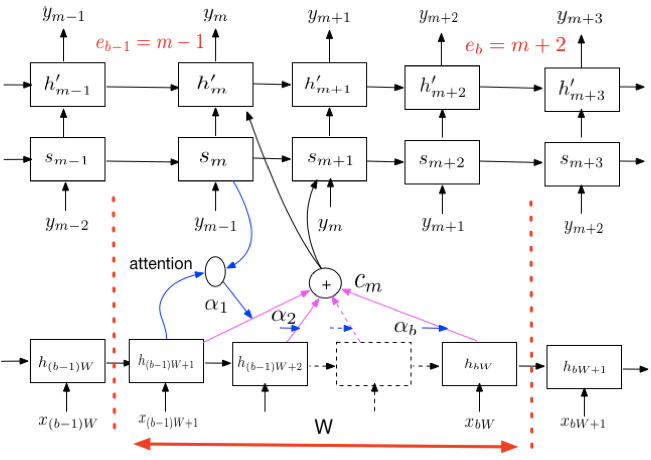Fig 10: Neural Transducer — attending to a limited part of the sequence. From this paper by Jaitly et al

Area Attention

Refer back to Fig 1, an illustration of the base introductory attention model we saw in the earlier post. A generalized abstraction of alignment is that it is like querying the memory as we generate the output. The memory is some sort of representation of the input and the query is some sort of representation of output. In Fig 1, the memory or collection of keys was the encoder hidden states “h”, the blue nodes, and query was the current decoder hidden state “s”, the green nodes. The derived alignment score is then multiplied with “values” — another representation of the input, the gold nodes in Fig 1.

Area attention is when attention is applied on to an “area”, not necessarily just one item like a vanilla attention model. “Area” is defined as a group of structurally adjacent items in the memory (i.e. the input sequence in a one dimensional input like sentence of words). An area is formed by combining adjacent items in the memory. In 2-D case like an image, the area will be any rectangular subset within the image.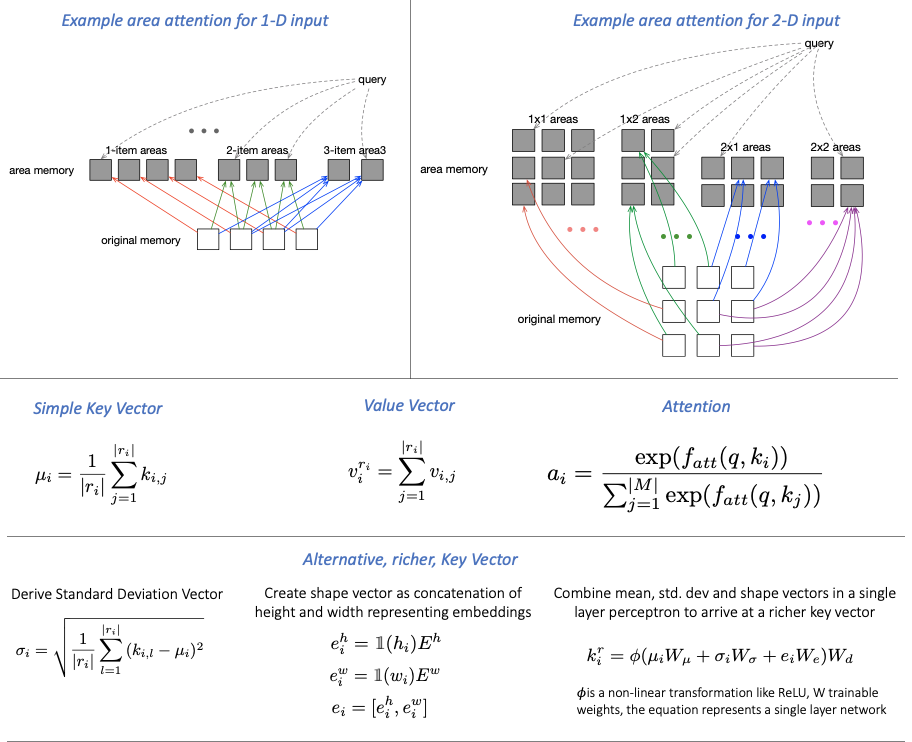Fig 11: Area attention from this paper by Yang et al.

The “key” vector for an area can be defined simply as the mean vector of the key of each item in the area. In a sequence to sequence translation task, this would be the mean of each of the hidden state vectors involved in the area. In the definition under “Simple Key Vector” in Fig 11, ”k” is the hidden state vector. If we are defining an area containing 3 adjacent words, then the mean vector is the mean of the hidden state vectors generated after each of the three words in the encoder.

The “value” on the other hand is defined as the sum of all value vectors in the area. In our basic example, this will again be the encoder hidden state vectors corresponding to the three words for which the area is being defined.

We can also define a richer representation of the key vector that takes into consideration not just the mean, but also the standard deviation and shape vector as explained in the Fig 11. Shape vector here is defined as the concatenation of height and width vectors, which in turn are created from actual width and height numbers projected as vectors using embedding matrices, which I presume are learnt with the model. The key is derived as an output of a single layer perceptron that takes mean, std dev and shape vectors as input.

Once the key and value vectors are defined, the rest of the network could be any attention utilizing model. If we are using a encoder-decoder RNN as seen in Fig 1, then plugging the derived area based key and value vectors in place of those in Fig 1 will make it an area based attention model.

Reading through these papers gives an interesting perspective on how researchers have used attention mechanisms for various tasks and how the thinking has evolved. Hopefully this quick study gives a sense of how we could tweak and use one of these or a new variant in our own tasks.